#### 期刊菜单

Fractality of Pore Structure in Cement Based Materials
DOI: 10.12677/HJCE.2022.116087, PDF, 下载: 91  浏览: 153

Abstract: Starting from the common mercury intrusion porosimetry test, the fractality in cement based materials is examined, which might be the pore mass fractal, the pore surface fractal or the solid mass fractal. The results indicate that the MIP test reveals the most probable fractal type being the solid mass fractal, and meanwhile the fractal dimension depends on the scale of interest. Moreover, a general approach of the solid mass fractal model is proposed to simulate pore structure in cement based materials.

1. 引言

2. 实验

$P=\frac{4\gamma \mathrm{cos}\theta }{d}$ (1)

$\gamma$ 是水银的表面张力，θ是水银和孔隙表面的接触角。本文 $\gamma$θ分别取为0.48 N/m和140˚ 。被测样品的孔隙尺寸分布(Pore size distribution, PSD)采用半对数坐标系，即dV/dlogd vs. logd。如图2所示，三组水泥浆样品孔隙尺寸大都在100 nm以下，即logd < 2。矿渣水泥浆的孔隙分布集中于0.5 < logd < 1.5。普通水泥浆和石灰水泥浆的孔隙分布集中于1 < logd < 2。究其原因，由于Ca(OH)2溶液养护的作用，矿渣水泥浆中矿渣组分的水化反应更为充分，不但降低了整体孔隙率而且细化了孔隙结构。Table 1. Mix proportion of cement pastesFigure 1. Porosimetric data of cement pastes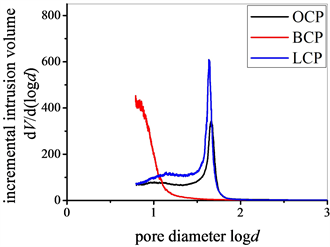Figure 2. Pore size distribution of cement pastes

3. 分形模式

Ji等定义变量V*表示在压汞实验中的反向累计压入量如下

${V}^{*}={\int }_{{d}_{\mathrm{min}}}^{d}\text{d}V$ (2)

dmin表示最小孔隙尺寸。如果V*和孔隙尺寸d满足式(3)，则属于孔隙分形。

${V}^{*}\propto {d}^{3-{D}_{PM}}$ (3)

DPM表示相应的孔隙分形维数。

$S=-\frac{1}{\gamma \mathrm{cos}\theta }{\int }_{0}^{V}P\text{d}V$ (4)

$S\propto {d}^{2-{D}_{PS}}$ (5)

DPS表示相应的孔隙–固相界面分形维数。

Usteri等定义变量 $\chi$ 表示在压汞实验中测得的密实度如下

$\chi =\frac{{V}_{\text{total}}-V}{{V}_{\text{total}}}$ (6)

Vtotal表示孔隙部分和固相部分的总体积。如果 $\chi$ 和孔隙尺寸d满足式(7)，则属于固相分形。

$\chi \propto {d}^{3-{D}_{SM}}$ (7)

DSM表示相应的固相分形维数。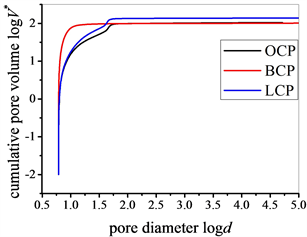(a)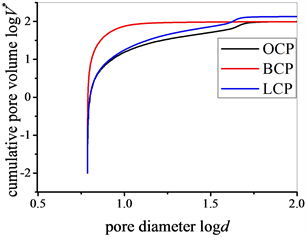(b)

Figure 3. Examining the property of pore mass fractal (a) 0.5 < logd < 5; (b) 0.5 < logd < 2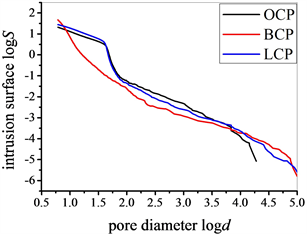(a)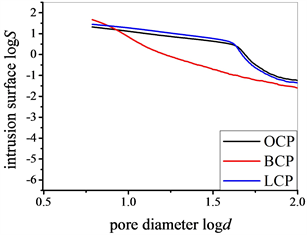(b)

Figure 4. Examining the property of pore surface fractal (a) 0.5 < logd < 5; (b) 0.5 < logd < 2Table 2. The property of solid mass fractal in different pore ranges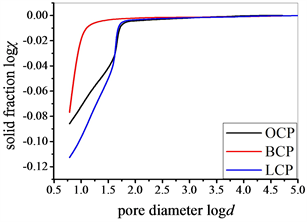(a)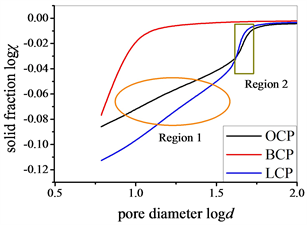(b)

Figure 5. Examining the property of solid mass fractal (a) 0.5 < logd < 5; (b) 0.5 < logd < 2Table 3. The property of solid mass fractal in different pore ranges

4. 分形模型

${\chi }_{i}={\left(\frac{{a}_{i}}{L}\right)}^{E-D}$ (8)Figure 6. Schematic illustration of the solid mass fractal model with E = 2, n = 3, and b = 5

5. 案例分析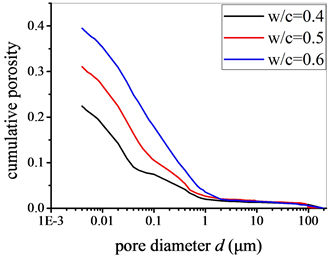Figure 7. MIP test data of ordinary cement pastes with varying w/c ratios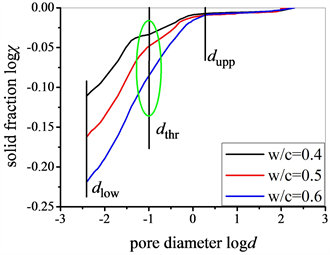Figure 8. The property of solid mass fractal for ordinary cement pastes with varying w/c ratiosTable 4. Structural parameters of ordinary cement pastes with varying w/c ratiosTable 5. Parameters of solid mass fractal model of ordinary cement pastes with varying w/c ratios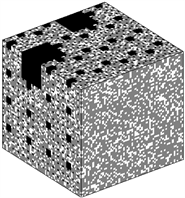(a)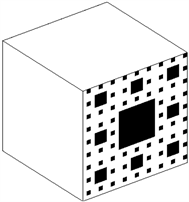(b)

Figure 9. Modeled pore structure of ordinary cement paste with w/c of 0.4 and curing age of 28 d (a) capillary pores; (b) C-S-H gel pores, where white denotes solid component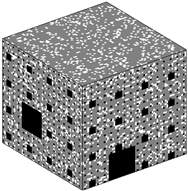(a)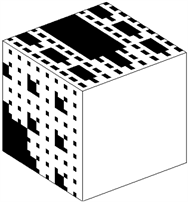(b)

Figure 10. Modeled pore structure of ordinary cement paste with w/c of 0.5 and curing age of 28 d (a) capillary pores; (b) C-S-H gel pores, where white denotes solid component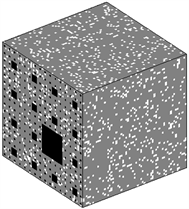(a)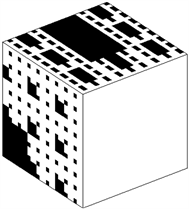(b)

Figure 11. Modeled pore structure of ordinary cement paste with w/c of 0.6 and curing age of 28 d (a) capillary pores; (b) C-S-H gel pores, where white denotes solid component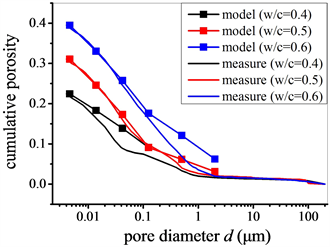Figure 12. Comparison of pore size distribution between measure and model

6. 结论

1) 水泥基材料多孔结构具有固相分形特征，且具有尺度依赖性。

2) 基于固相分形的一般构造方法，硬化水泥浆多孔结构的模拟按照孔隙尺寸从大(毛细孔)到小(凝胶孔)逐级进行。

NOTES

*通讯作者。

  Arandigoyen, M., Perez Bernal, J.L., Bello Lopez, M.A., et al. (2005) Lime-Pastes with Different Kneading Water: Pore Structure and Capillary Porosity. Applied Surface Science, 252, 1449-1459. https://doi.org/10.1016/j.apsusc.2005.02.145  Gimenez, D., Perfect, E., Rawls, W.J., et al. (1997) Fractal Models for Predicting Soil Hydraulic Properties: A Review. Engineering Geology, 48, 161-183. https://doi.org/10.1016/S0013-7952(97)00038-0  Perez Bernal, J.L. and Bello, M.A. (2001) Fractal Geometry and Mercury Porosimetry. Comparison and Application of Proposed Models on Building Stones. Applied Surface Science, 185, 99-107. https://doi.org/10.1016/S0169-4332(01)00649-3  Atzeni, C., Pia, G. and Sanna, U. (2008) Fractal Modelling of Medium-High Porosity SiC Ceramics. Journal of the European Ceramic Society, 28, 2809-2814. https://doi.org/10.1016/j.jeurceramsoc.2008.03.039  Mandelbrot, B.B. (1983) The Fractal Geometry of Nature. WH Freeman, New York. https://doi.org/10.1119/1.13295  Jennings, H.M., Bullard, J.W., Thomas, J.J., et al. (2008) Characterization and Modeling of Pores and Surfaces in Cement Paste: Correlations to Processing and Properties. Journal of Advanced Concrete Technology, 6, 5-29. https://doi.org/10.3151/jact.6.5  Lange, D.A., Jennings, H.M. and Shah, S.P. (1994) Image Analysis Techniques for Characterization of Pore Structure of Cement-Based Materials. Cement and Concrete Research, 24, 841-853. https://doi.org/10.1016/0008-8846(94)90004-3  Winslow, D.N., Bukowski, J.M. and Young, J.F. (1995) The Fractal Arrangement of Hydrated Cement Paste. Cement and Concrete Research, 25, 147-156. https://doi.org/10.1016/0008-8846(94)00122-F  Ji, X., Chan, S.Y.N. and Feng, N. (1997) Fractal Model for Simulating the Space-Filling Process of Cement Hydrates and Fractal Dimensions of Pore Structure of Cement-Based Materials. Cement and Concrete Research, 27, 1691-1699. https://doi.org/10.1016/S0008-8846(97)00157-9  Zeng, Q., Li, K.F., Chong, T.F., et al. (2010) Surface Fractal Analysis of Pore Structure of High-Volume Fly-Ash Cement Pastes. Applied Surface Science, 257, 762-768. https://doi.org/10.1016/j.apsusc.2010.07.061  Chen, H., Wyrzykowski, M., Scrivener, K., et al. (2013) Prediction of Self-Desiccation in Low Water-to-Cement Ratio Pastes Based on Pore Structure Evolution. Cement and Concrete Research, 49, 38-47. https://doi.org/10.1016/j.cemconres.2013.03.013  Neimark, A.V. (1992) A New Approach to the Determination of the Surface Fractal Dimension of Porous Solids. Physica A, 191, 258-262. https://doi.org/10.1016/0378-4371(92)90536-Y  Usteri, M., Bonny, J.D. and Leuenberger, H. (1990) Fractal Dimension of Porous Solid Dosage Forms. Pharmaceutica Acta Helvetiae, 65, 55-61.  Ridi, F., Fratini, E. and Baglioni, P. (2013) Fractal Structure Evolution during Cement Hydration by Differential Scanning Calorimetry: Effect of Organic Additives. The Journal of Physical Chemistry C, 117, 25478-25487. https://doi.org/10.1021/jp406268p  Gao, Y., Wu, K. and Jiang, J.Y. (2016) Examination and Modeling of Fractality for Pore-Solid Structure in Cement Paste: Starting from the Mercury Intrusion Porosimetry Test. Construction and Building Materials, 124, 237-243. https://doi.org/10.1016/j.conbuildmat.2016.07.107  Tang, S.W., He, Z., Cai, X.H., et al. (2017) Volume and Surface Fractal Dimensions of Pore Structure by NAD and LT-DSC in Calcium Sulfoaluminate Cement Paste. Construction and Building Materials, 143, 395-418. https://doi.org/10.1016/j.conbuildmat.2017.03.140  Zhang, L. and Zhou, J. (2020) Fractal Characteristics of Pore Structure of Hardened Cement Paste Prepared by Pressurized Compact Molding. Construction and Building Materials, 259, Article ID: 119856. https://doi.org/10.1016/j.conbuildmat.2020.119856  Gao, Y., Wu, K., Rong, Z.D., et al. (2021) A Hybrid Analytical-Numerical Algorithm Based General Modeling Framework of Molecular Diffusivity in Cement Paste. International Journal of Heat and Mass Transfer, 180, Article ID: 121774. https://doi.org/10.1016/j.ijheatmasstransfer.2021.121774